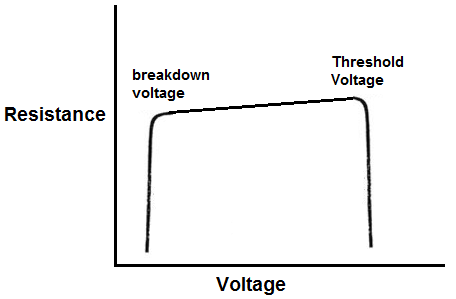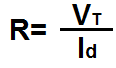﻿ Diode Resistance- Explained ﻿# Diode ResistanceDiode resistance is the resistance which a diode offers in a circuit.

Just like a resistor or any other load in a circuit, a diode offers resistance in a circuit.

Unlike resistors, though, diodes are not linear devices. This means that the resistance of diodes does not vary directly and proportional to the amount of voltage and current applied to them. It changes parabolically.

Below is a graph of the resistance of a resistor or any other linear load:

### Resistor Resistance GraphAs you can based on the graph of a resistor, the resistance is constant. It does not vary when either voltage or current is changed in a circuit. When you have a 10KΩ resistor in a circuit, it will offer 10KΩ resistance in the circuit regardless of the voltage or current of the circuit.

The resistance can be calculated by the formula,R=V/I.

Diodes, however, do not work like this. Diodes are not linear devices; they are nonlinear. Since diodes are semiconductor devices doped with impurities, they do not function like linear devices. Their resistance changes based on the voltage and current that falls across them. Resistance is not constant.

And diode resistance does not change in a linear sense, but in a parabolic sense.

Below is a graph of diode resistance:

### Diode Resistance GraphAs you can see with diodes, resistance changes parabolically.

The diode has 2 key resistance change periods, at the breakdown voltage and at the threshold voltage.

Resistance does not change much but after the diode reaches the threshold voltage, resistance just decreases drastically, allowing a great amount of current to rapidly pass through the diode. The threshold voltage is the voltage where the diode has enough voltage to conduct a large amount of current through it. Without this threshold voltage, the diode does not have enough power to conduct current. The threshold voltage is normally 0.7V for silicon diodes and 0.3V for germanium diodes.

The same occurs to the left. Resistance does not change much until the diode reaches the breakdown voltage. As this point, the resistance drastically decreases, allowing a great deal of current to flow through. The breakdown voltage is the voltage which the diode has received the maximum reverse voltage that a diode can withstand. If more voltage is fed above this point, the diode will conduct a large amount of current across its terminals. Diodes are meant to only pass current when forward biased. But if enough reverse voltage is applied to it, its breakdown point, it will also conduct current across its junctions in reverse.

### Diode Resistance Formula

The resistance of diodes is equal to the below formula:Diode resistance is equal to the thermal voltage, VT, divided by the current, Id, passing through the diode.

The thermal voltage of the diode is approximately 25mV at 300K, which is a temperature that is very close to room temperature.

The precise formula to calculate thermal voltage is:

VT= kT/q

where k is the Boltzmann constant, T is the absolute temperature of the pn junction, and q is the magnitude of the electron charge.

You can calculate the thermal voltage in this way, but for all practical purposes, 25mV should be substituted for the thermal voltage.

The current passing through the diode can be calculated according to the formula:This formula can be used to calculate diode current.

If you have a circuit actually hooked up to a breadboard, then you can just use a multimeter turned to the ammeter setting. Connect the probes in series with the circuit and then read the amount of current. You can then use this value in the above formula to calculate the resistance.

Once the thermal voltage and the diode current are calculated, the diode resistance can be calculated using the formula, Rd= VT/ID.

Related Resources